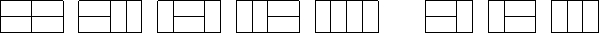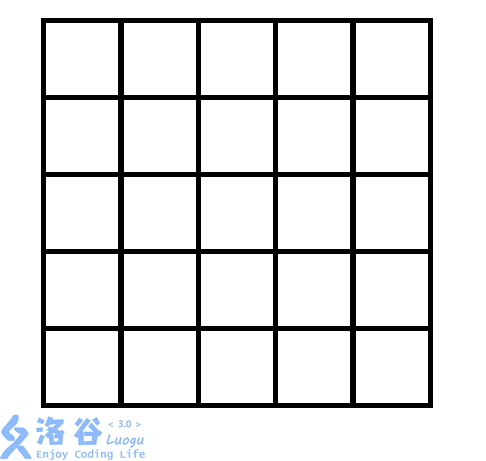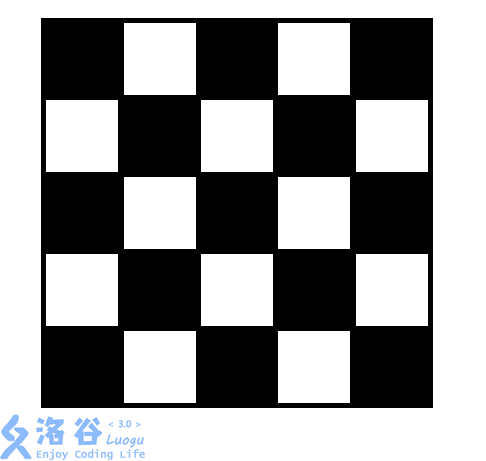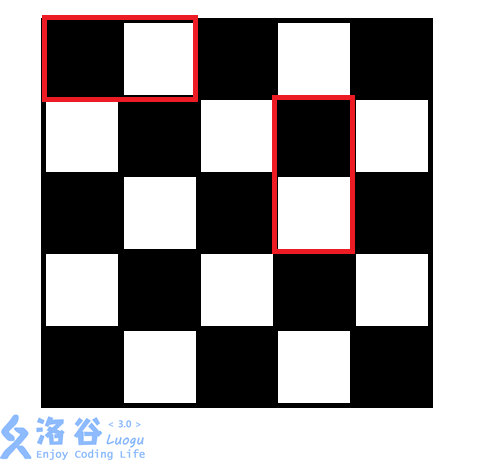Mondriaan 的梦（HG 2018.4.15 试题1 T3）

2018-04-19 11:51:14

## 题目部分

### 【题目描述】2 4

5

## 题解部分

1. 判断第i位是否为0 -> (s & 1 << i) == 0，意思是将1左移i位与s进行与运算后，看结果是否为0.
2. 将第i位置变为1 -> s | 1 << i，意思是将1左移动i位与s进行或运算.
3. 将第i位置变为0 -> s & !(1 << i)，意思是s与第i位为0，其余位为1的数进行与运算。
#include<cstdio>
#include<cstring>
#include<iostream>
#include<algorithm>
using namespace std;
int n,m;
long long f[1<<15];
void dfs(int i,int j,int s,int p)
{
if(p==m)
{
f[i][j]+=f[i-1][s];
return;
}
if((1<<p)&j) dfs(i,j,s,p+1);
else
{
dfs(i,j,(s|(1<<p)),p+1);
if(p+2<=m && !(j&(1<<(p+1)))) dfs(i,j,s,p+2);
}
}
int main()
{
scanf("%d%d",&n,&m);
if(n%2 && m%2)//特判，都为奇数无法填满，不加也可以
{
cout<<0;
return 0;
}
f=1;
for(int i=1;i<=n+1;i++)
for(int j=0;j<(1<<m);j++)
dfs(i,j,0,0);
cout<<f[n+1];
return 0;
}

1.总方格数为奇数，而两个两个填总数必为偶数，矛盾

2.牛逼的染色法，将图进行奇偶染色如下图• star
首页